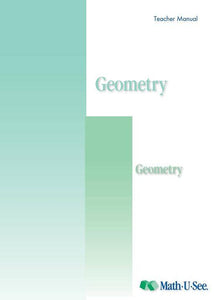or 6 weekly interest-free payments from \$14.83 withwhat's this?Demme Learning

# Math.U.See Geometry

Regular price \$20.00 \$50.83 Unit price per
Tax included. Shipping calculated at checkout.

## Math.U.See Geometry

#### Points, lines, planes, angles, circles, triangles, quadrilaterals, Pythagorean Theorem, conic sections, proofs and more.

Major Concepts and Skills Include:

• Describing points, lines, rays, line segments, angles, and planes
• Calculating the measure of the interior and exterior angles of a regular polygon
• Understanding the geometry of a circle, sphere, and ellipse
• Understanding and computing volume and surface area of solids
• Using the Pythagorean theorem to identify triangle attributes
• Applying postulates, theorems, definitions, and properties to geometric proofs

• Using a protractor to construct angles
• Using a compass to construct bisectors
• Constructing and identifying triangles
• Working with algebraic expressions containing radicals
• Completing geometric transformations within a Cartesian plane
• Understanding basic trigonometric functions

Geometry Instruction Pack includes: Instruction Manual & DVD

Geometry Student pack includes: Student Workbook & Test & Activity booklet.

Honours Textook:  Geometry Honors Textbook provides extension work or extra practice for those working through Geometry.

Grab an extra Student Pack for an additional Student.